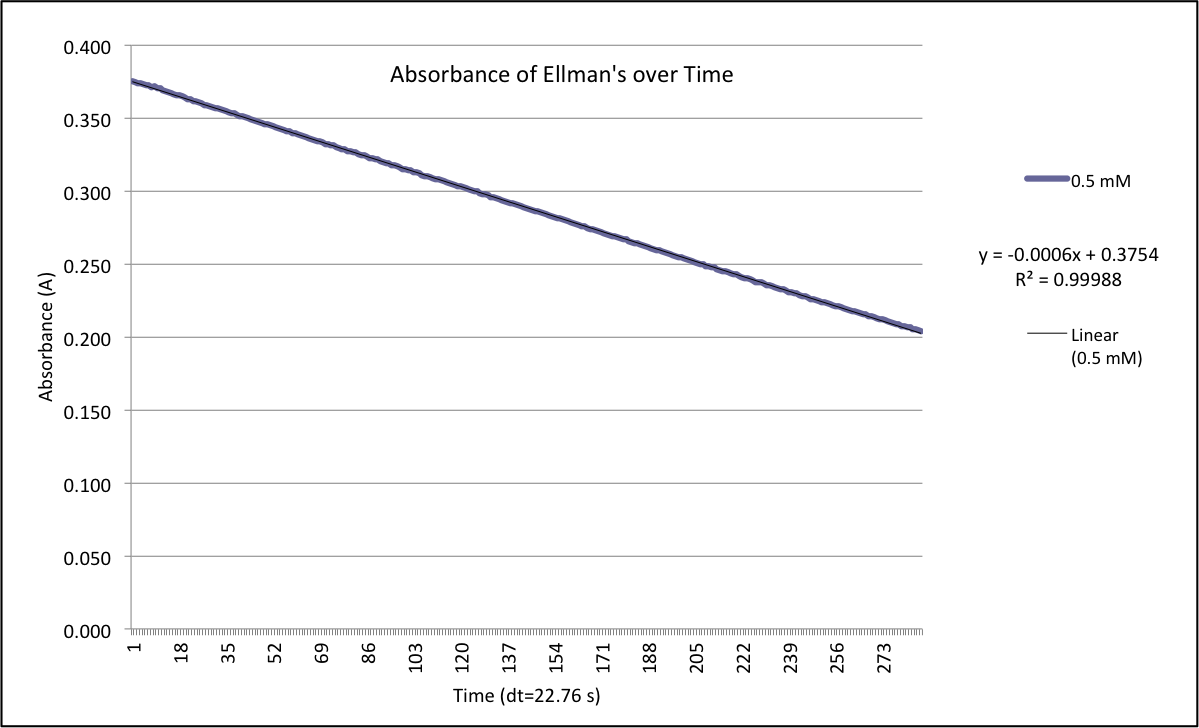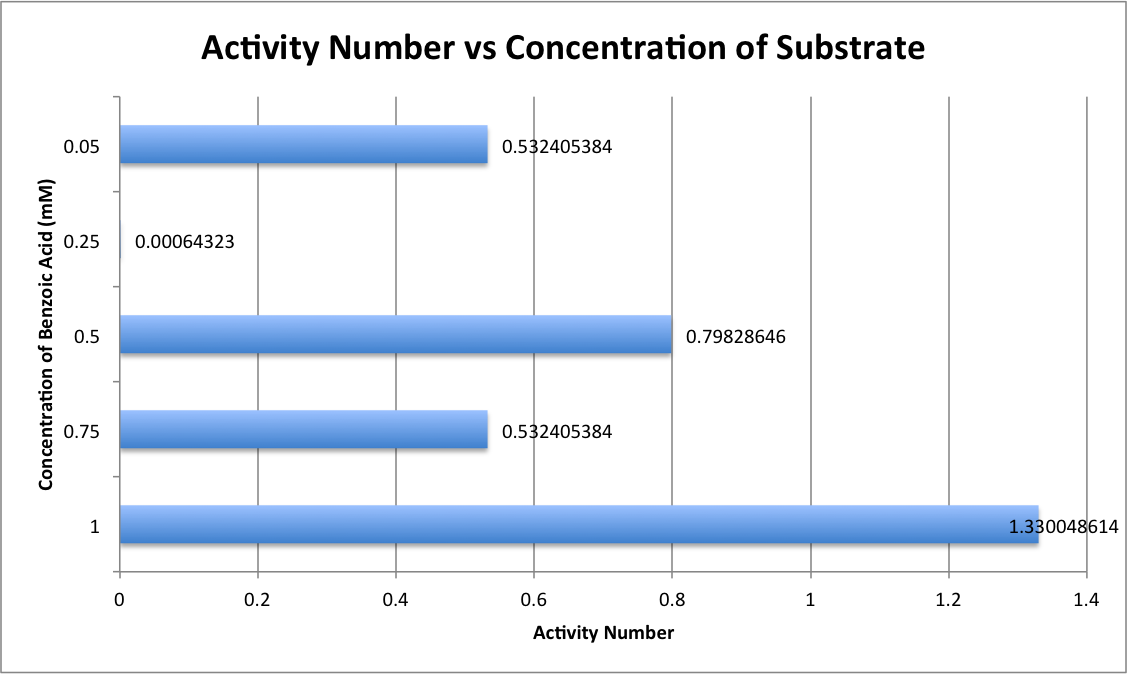# Team:Duke/Results

In order to characterize our enzyme, it was necessary to determine activity rate of the introduced enzyme. This was done by graphing the data from the BadA lysate and the Empty Vector lysate (see graph below), in order to normalize for potential endogenous reactions. In the graph below, it is possible to see that the 412 nm absorption of the BadA lysate decreased farther and at a greater rate than the absorption of the Empty Vector lysate. These results indicate that the enzyme is actively converting the substrates to benzoyl-CoA.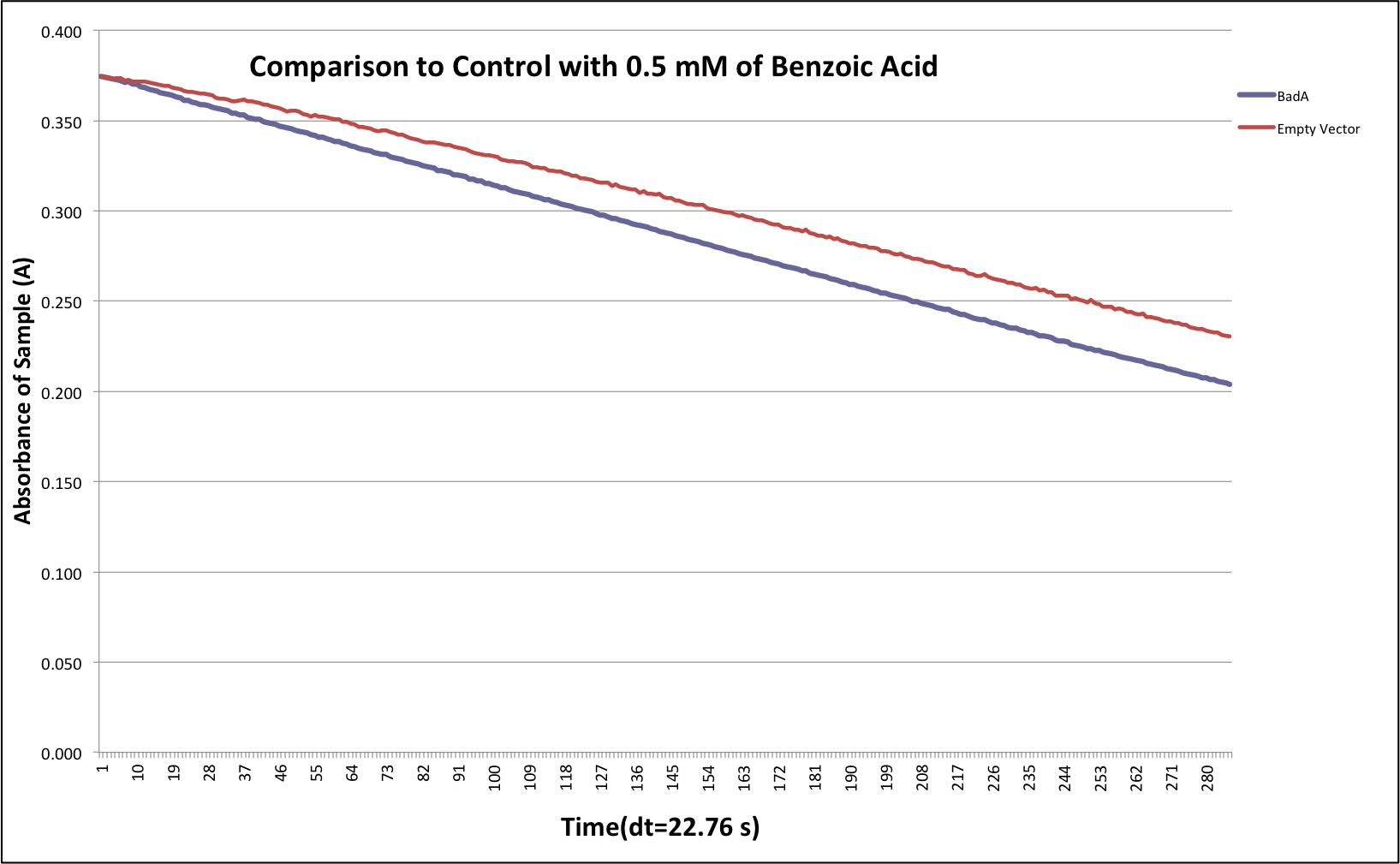Another part of determining if the test was valid was to compare the controls to the sample of interest. This was done by graphing the samples from the above graph with their respective controls (see the following two graphs). These graphs indicate that the CoA was being registered as the trial without CoA added did not have any absorbance, meaning the lysate contained little endogenous CoA. We can also determine that the cells were active because the samples without ATP or benzoic acid were reacting a decrease rate compared to the complete substrate sample. Overall, these trials confirmed that the rate of decrease for for the enzymatic reaction exceed any background reactions.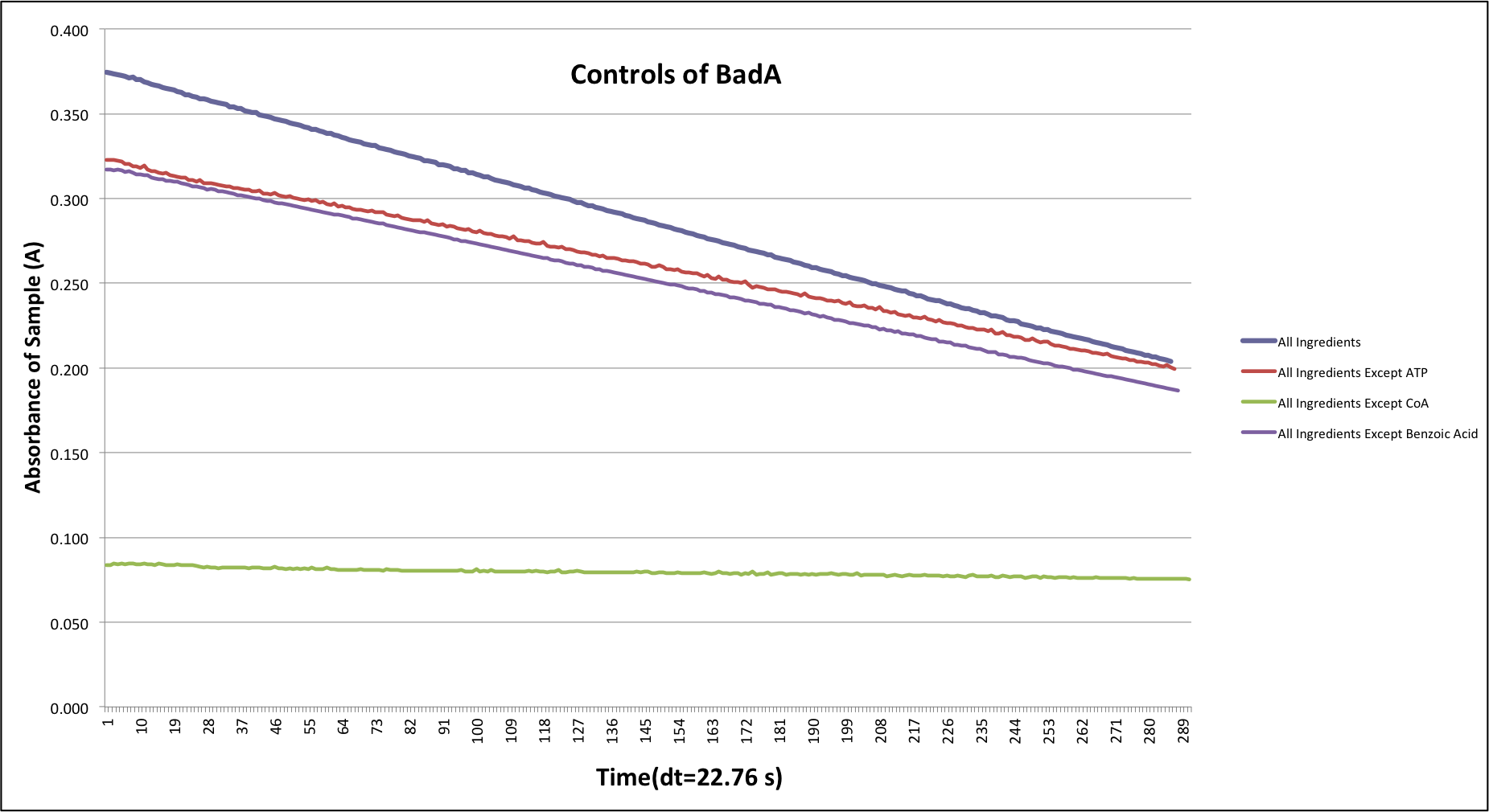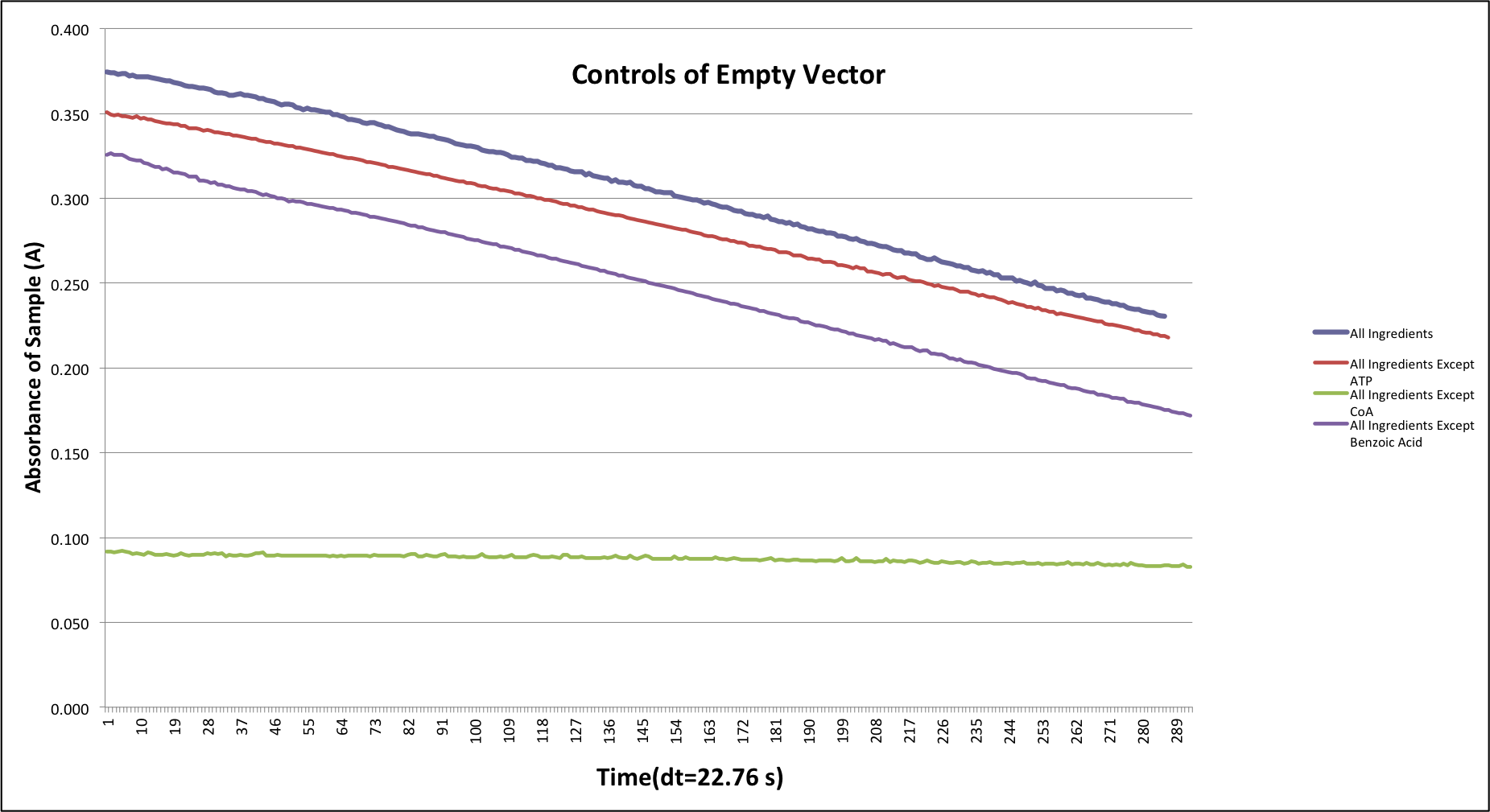## Varying the Amount of Benzoic Acid

After confirming that the BadA enzyme is working, it is necessary to investigate the absorption of the Ellman’s Reagent when the amount of benzoic acid was varied. In the experiment the benzoic acid was varied through the following values: 0.05, 0.25, 0.5, 0.75, and 1 mM. The graph of these trials can be seen in the graph below.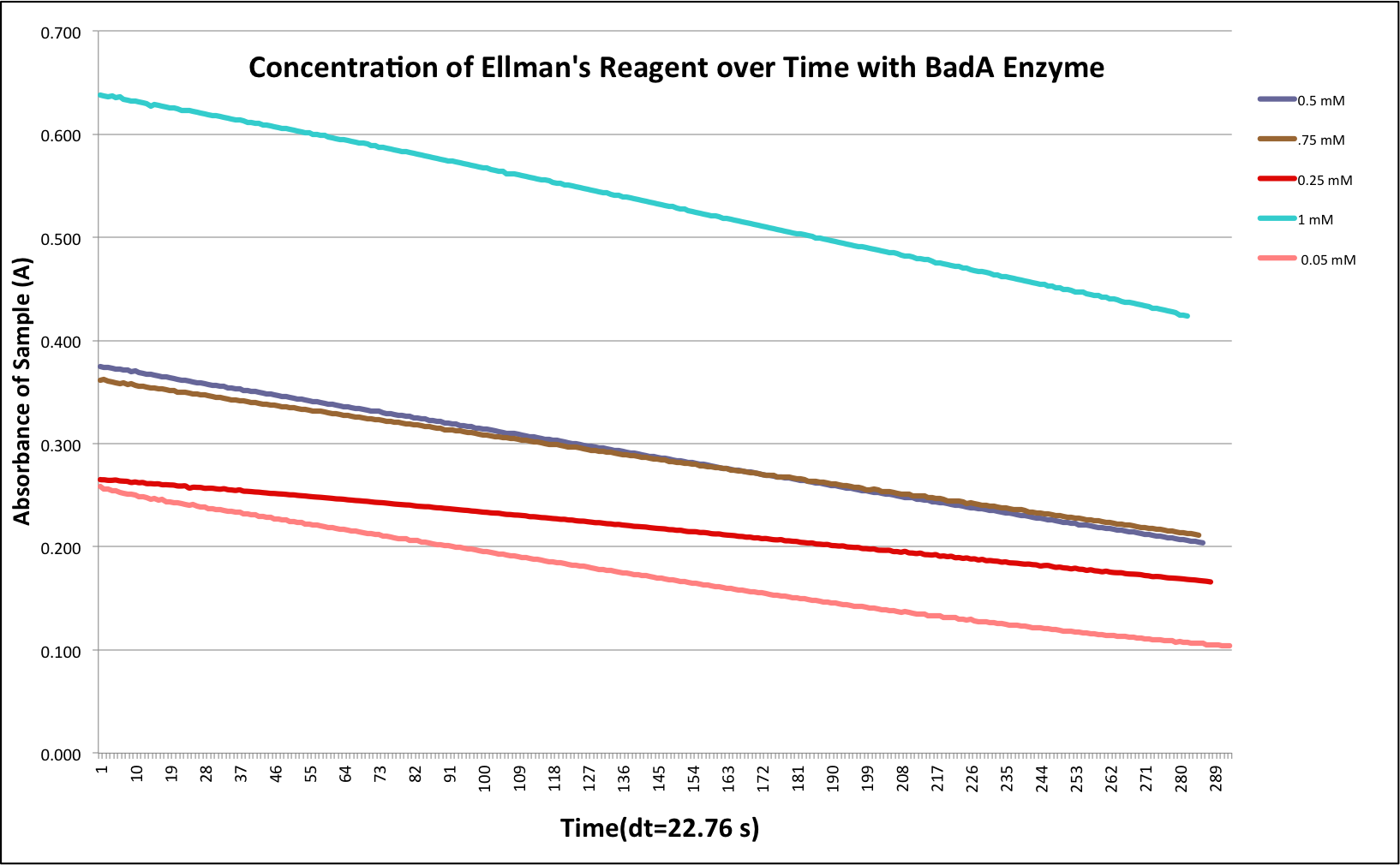## Activity Number

Using the data from the graph above, the slope of the graph was determined. See the graph below for an example on how the slope of these graphs were determined. Once the slope was determined, the activity number for the enzyme could be calculated. The activity number was calculated by taking the slope and dividing by the extinction coefficient (which is divided by the path length) and then normalizing the number by the amount of protein in the sample. The activity numbers were then normalized by the activity number for the empty vector control. The resulting activity numbers can be seen in the figure below.